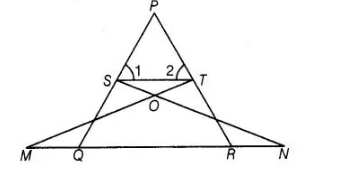# In figure, if ∠1 =∠2 and ΔNSQ = ΔMTR,

Question:

In figure, if ∠1 =∠2 and ΔNSQ = ΔMTR, then prove that ΔPTS ~ ΔPRQ.Solution:

Given $\triangle N S Q \cong \triangle M T R$ and $\angle 1=\angle 2$

To prove $\triangle P T S \sim \Delta P R Q$

Proof Since, $\triangle N S Q \cong \triangle M T R$

So, $S Q=T R$ ...(i)

Also, $\angle 1=\angle 2 \Rightarrow P T=P S$ ...(ii)

[since, sides opposite to equal angles are also equal]

From Eqs. (i) and (ii), $\frac{P S}{S Q}=\frac{P T}{T R}$

$\Rightarrow$ $S T \| Q R$ [by convense of basic proportionality theorem]

$\therefore$ $\angle 1=\angle P Q R$

and $\angle 2=\angle P R Q$

In $\triangle P T S$ and $\triangle P R Q$, [common angles]

$\angle P=\angle P$

$\angle 1=\angle P Q R$

$\angle 2=\angle P R Q$

$\therefore$ $\Delta P T S \sim \Delta P R Q$ [by AAA similarity criterion]

Hence proved.